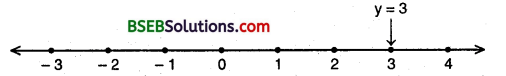# Bihar Board Class 9th Maths Solutions Chapter 4 Linear Equations in Two Variables Ex 4.4

Bihar Board Class 9th Maths Solutions Chapter 4 Linear Equations in Two Variables Ex 4.4 Textbook Questions and Answers.

## BSEB Bihar Board Class 9th Maths Solutions Chapter 4 Linear Equations in Two Variables Ex 4.4Question 1.
Give the geometric representations of y = 3 as an equation
(i) in one variable
(ii) in two variables
Solution:
(i) The representation of the solution on the number when y = 3 is treated as an equation in one variable is as under(ii) We know that y = 3 can, be written as 0.x + y = 3 as a linear equation in variables x and y. Now all the values of x are permissible as 0.x is always 0. However, y must satisfy the relation y = 3.
Hence, three solution of the given equation are
x = 0, y = 3;
x = 2, y = 3Plotting the points (0, 3), (2, 3) and (- 2, 3) and on joining them we get the graph AB as a line parallel to x-axis at a distance of 3 units above it.Question 2.
Give the geometric representations of 2x + 9 = 0 as an equation
(i) in one variable
(ii) in two variables
Solution:
(i) The representation of the solution on the number line 2x + 9 = 0 i.e., x = – $$\frac { 9 }{ 2 }$$ is treated as an equation in one variable is as under(ii) We know that 2x + 9 = 0 can be written as 2x + O.y + 9 = 0 as a linear equation in variables x and y. Now all the values of x are permissible as O.y is always 0. However, x must satisfy the relation 2x + 9 = 0 i.e., x = – $$\frac { 9 }{ 2 }$$
Hence three solutions of the given equation are x = – $$\frac { 9 }{ 2 }$$
y = 0; x = – $$\frac { 9 }{ 2 }$$, y = 2 and x = – $$\frac { 9 }{ 2 }$$, y = – 2.
∴ Plotting the points (- $$\frac { 9 }{ 2 }$$, 0), (- $$\frac { 9 }{ 2 }$$, 2) and (- $$\frac { 9 }{ 2 }$$, 2) and on joining them we get the graph AB as a line parallel to y-axis at a distance of $$\frac { 9 }{ 2 }$$ on the left of y-axis.